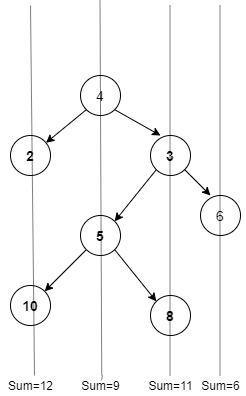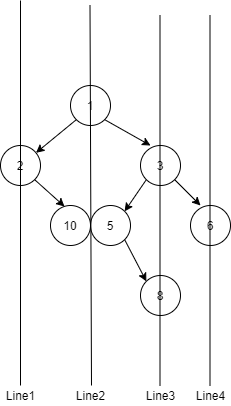1

# Vertical Sum in BST

Difficulty: MEDIUMContributed By
Hardik Aggarwal
Avg. time to solve
25 min
Success Rate
75%

Problem Statement
Suggest Edit

#### You are required to print the sum along vertical lines from the leftmost node and moving to the rightmost node.

##### For Example :
``````Consider the following Binary Tree:
````````````So the final answer is
12 9 11 6
``````
##### Input Format :
``````The first line of input contains an integer ‘T’ denoting the number of test cases.
The next ‘T’ lines represent the ‘T’ test cases.

The first line of each test case contains the elements of the tree in the level order form separated by a single space. If any node does not have a left or right child, take -1 in its place.
For example, the input for the tree depicted in the below image would be :
````````````1
2 3
4 -1 5 6
-1 7 -1 -1 -1 -1
-1 -1
``````

#### Explanation :

``````Level 1 :
The root node of the tree is 1

Level 2 :
Left child of 1 = 2
Right child of 1 = 3

Level 3 :
Left child of 2 = 4
Right child of 2 = null (-1)
Left child of 3 = 5
Right child of 3 = 6

Level 4 :
Left child of 4 = null (-1)
Right child of 4 = 7
Left child of 5 = null (-1)
Right child of 5 = null (-1)
Left child of 6 = null (-1)
Right child of 6 = null (-1)

Level 5 :
Left child of 7 = null (-1)
Right child of 7 = null (-1)

The first not-null node (of the previous level) is treated as the parent of the first two nodes of the current level. The second not-null node (of the previous level) is treated as the parent node for the next two nodes of the current level and so on.
The input ends when all nodes at the last level are null (-1).
``````
##### Note :
``````The above format was just to provide clarity on how the input is formed for a given tree.
The sequence will be put together in a single line separated by a single space. Hence, for the above-depicted tree, the input will be given as:

1 2 3 4 -1 5 6 -1 7 -1 -1 -1 -1 -1 -1
``````
##### Output Format :
``````For each test case, print the vertical sum of node values separated by a space in a separate line.
``````
##### Constraints :
``````1 <= T <= 10
1 <= N <= 3000
1 <= node data <= 10^9

Time Limit: 1sec
``````
##### Note :
``````You do not need to print anything, it has already been taken care of. Just implement the given function.
``````

#### Sample input 1:

``````2
1 2 3 -1 10 5 6 -1 -1 -1 8 -1 -1 -1 -1
20 8 22 5 3 -1 -1 -1 -1 -1 -1
``````

#### Sample output 1:

``````2 16 11 6
5 8 23 22
``````

#### Explanation of sample input 1:

``````For the first test case:
````````````As shown in the above figure,
Nodes connected by Line 1 = 2, Sum=  2
Nodes connected by Line 2= 1,5,10 Sum = 16  (1+5+10)
Nodes connected by Line 3=  3,8, Sum =11  (3+8)
Nodes connected by Line 4= 6, Sum= 6

2 16 11 6

For the second test case :

Nodes connected by Line 1 = 5 Sum=  5
Nodes connected by Line 2 = 8 Sum = 8
Nodes connected by Line 3 =20,3 Sum =23 (20+3)
Nodes connected by Line 4 =  22  Sum= 22

5 8 23 22
``````

#### Sample input 2:

``````2
1 2 3 4 -1 6 7 -1 -1 -1 8 -1 -1 -1 -1
1 5 -1 6 -1 7 -1 -1 -1
``````

#### Sample output 2:

``````4 2 7 11 7
7 6 5 1
``````Console